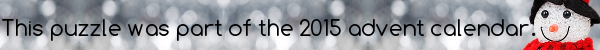mscroggs.co.uk
mscroggs.co.uksubscribe

# Advent calendar 2015

## 19 December1 = 1/2 + 1/4 + 1/8 + 1/16 + 1/16. This is the sum of 5 unit fractions (the numerators are 1).
In how many different ways can 1 be written as the sum of 5 unit fractions? (the same fractions in a different order are considered the same sum.)

## Archive

Show me a random puzzle
▼ show ▼
© Matthew Scroggs 2019